# Creating mean energy maps (aka pseudo temperature maps)## Overview

#### Synopsis:

The process for creating temperature maps by spectral fitting typically requires a significant amount of CPU time. This thread shows how to compute a map of the mean energy of the events which can done quickly and can help guide and inform users who want to then undertake the process spectral fitting. In short: the mean energy map can be thought of as a pseudo temperature map. Some authors, such as David et al.,( 2009, ApJ, 705, 624), have derived simple relationships for calibrating the mean energy with thermal models for specific objects; this is beyond the scope of this thread.

#### Purpose:

This thread can be run by users who may want to create a mean energy map and desire to get some quick results before investing time to perform spectral fits to create temperature maps.

Last Update: 05 Jan 2023 - First publication of this thread.

## Getting Started

In this thread we will looking at the spatial distribution of energy in OBS_ID 16014, a ~120 ksec observation of NGC7618.

`unix% download_chandra_obsid 16014 `

To remove the foreground point-like sources we will be following the basic steps An Image of Diffuse Emission thread. Users should refer to that thread for more details about specific steps shown below.

First we create the image, exposure map, and PSF map needed to run wavdetect to detect the point like sources. For this example thread we choose to restrict the data to just center chip, ACIS-7 aka ACIS-S3.

```unix% fluximage 16014/repro/acisf16014_repro_evt2.fits"[ccd_id=7]" out=ngc7618 \
bin=1 psfecf=0.5 mode=h clob+ verb=0
```

Next we run wavdetect

```unix% punlearn wavdetect wrecon wtransform
unix% pset wavdetect \
outfile=wav.src defn=wav.nbkg scell=wav.cell image=wav.recon \
scales="1.4 2 4 8 12"

unix% wavdetect mode=h clobber=yes
```

The sources are displayed in Figure 1. Overall the detection look fine, except that the core of diffuse emission was also detected. Since we want to keep those data we will be filtering out that detection in the next step.

### Figure 1: NGC 7618 broad band with source detections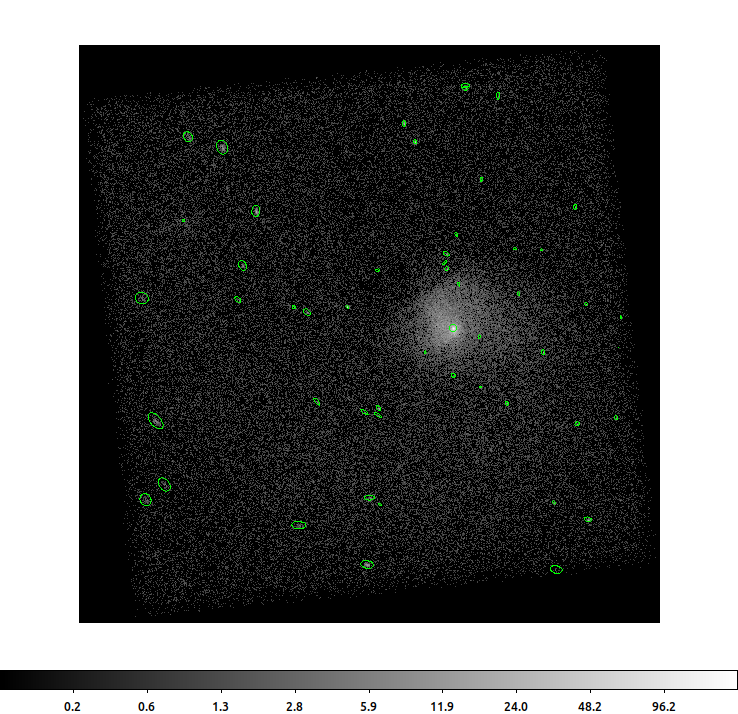### Figure 1: NGC 7618 broad band with source detections

The broad band, 0.5-7.0keV, image of NGC7618 shown with the wavdetect sources. The center of diffuse emission was also detected; that detection will need to be omitted in our analysis.

```unix% ds9 ngc7618_broad_thresh.img -block to 2 -region load wav.src
```

Next we stray slightly from the diffuse emission thread and will create different regions based on the wavdetect source list. We will use the psfsize_src tool to create circular source regions that enclose a specified fraction of the PSF. We choose to do this here (1) because the wavdetct source regions are highly irregular, and (2) it gives us the opportunity to filter out the source coincident with the core of the diffuse emission. This step is optional and one can proceed directly with the wavdetect source list if desired.

```unix% psfsize_srcs \
pos=wav.src"[exclude (x,y)=circle(3976,4057,2)]" \
outfile=psfsize.src ecf=0.9 energy=1.0 mode=h clob+
```

This creates circular regions that enclose ~90% of the PSF at 1.0keV. These regions are shown in Figure 2.

### Figure 2: NGC 7618 broad band with 90% ECF regions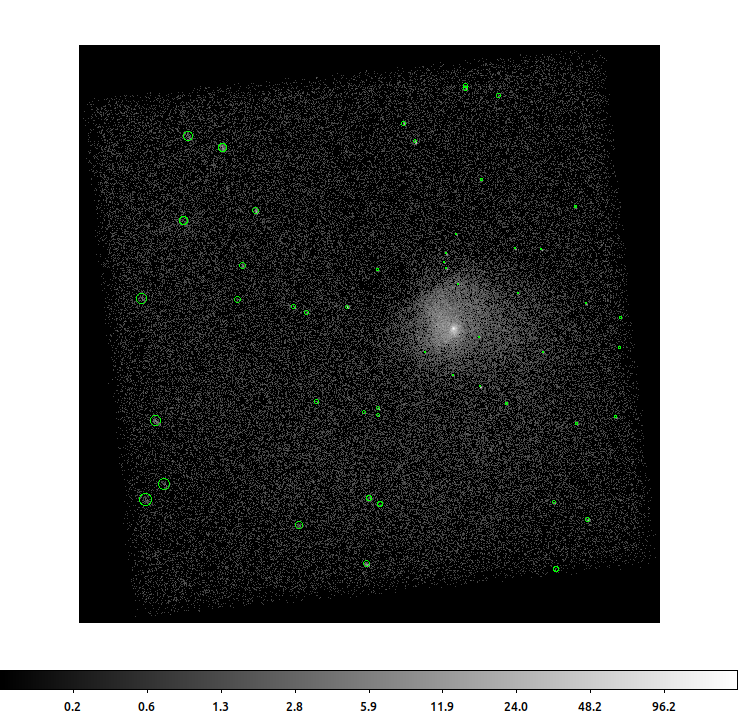### Figure 2: NGC 7618 broad band with 90% ECF regions

The same as Figure 1 but now with the psfsize_src regions shown. Note that the source coincident with the center of the diffuse emission has been removed.

```unix% ds9 ngc7618_broad_thresh.img -block to 2 -region load psfsize.src
```

Returning to the thread, the next step is to create source and background regions using the roi tool

```unix% mkdir regions

unix% punlearn roi
unix% roi infile=psfsize.src fovregion="region(ngc7618_16014.fov)" \
outsrc="regions/%04d.reg" \
compute- clob+ mode=h
```

This creates one output file for each source. Each file has two extensions: SRCREG, source region, and BKGREG, background region.

Upon closer inspection we see that we have two source regions that overlap. The sources are near the top-center of the image and their regions are shown in Figure 3. The logic used by roi when using group=exclude is that the overlapping area is excluded from both sources; the counts in the overlap area are not assign to either source.

### Figure 3: Overlapping source regions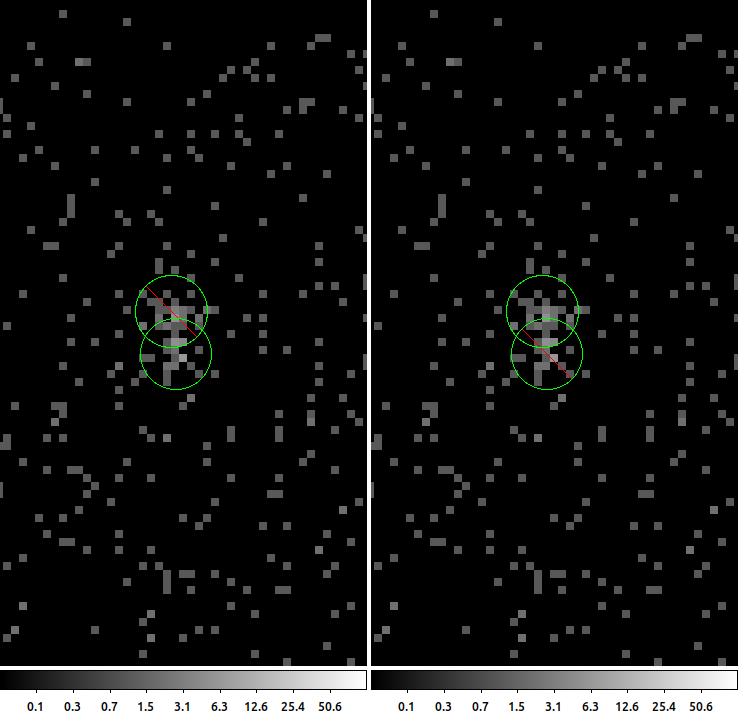### Figure 3: Overlapping source regions

This is a zoomed in image of the top-center of the data showing two overlapping sources. In the left frame we see that the top source is excluded from the bottom source; in the right frame we see that the bottom source is excluded from the top source. The result is that the overlapping area is not assigned to either source.

```unix% ds9 ngc7618_broad_thresh.img -zoom to 8 \
-pan to 4003 4540 physical \
-pan to 4003 4540 physical \
-tile column
```

For our purposes in this thread we want to be sure that the overlapping area is assigned to one of the sources since we want to fill in that area too. We use the rank_roi script to assign the overlapping area to the source with the maximum number of counts.

```unix% mkdir ranked_regions
unix% punlearn rank_roi

unix% rank_roi ngc7618_broad_thresh.img roi="regions/????.reg" out="ranked_regions/{:04d}.reg" method=max clob+
```

rank_roi creates a new set of region files where the overlapping area, if any, is assigned to only one of the sources. The output from rank_roi is shown in Figure 4.

### Figure 4: Overlap after ranking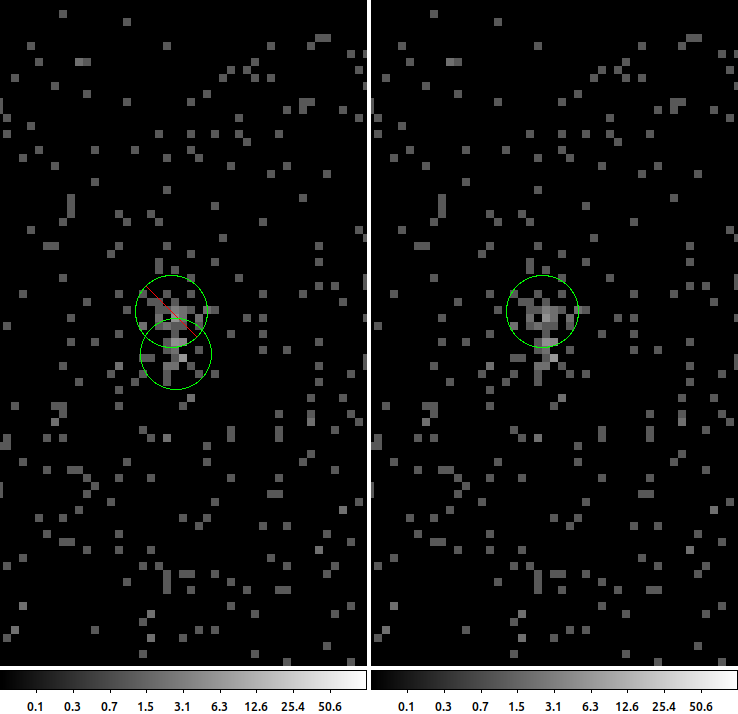### Figure 4: Overlap after ranking

This is same as Figure 3 but after the regions have been ranked based on the number of counts. The source with the maximum number of counts is assigned the overlapping area. The source shown on the left frame is unchanged; the top source is being excluded from the bottom source. However, now the source shown in the right frame no longer has the bottom source excluded; the events in the overlap have been assigned to top source.

```unix% ds9 ngc7618_broad_thresh.img -zoom to 8 \
-pan to 4003 4540 physical \
-pan to 4003 4540 physical \
-tile column
```

We can now create stacks of source and background regions

```unix% /bin/ls ranked_regions/????.reg | sed 's,.*,region(&[srcreg]),' > src.lis
unix% /bin/ls ranked_regions/????.reg | sed 's,.*,region(&[bkgreg]),' > bkg.lis
```

And then run dmfilth to remove the point-like sources

```unix% punlearn dmfilth
src=@src.lis bkg=@bkg.lis method=POISSON clob+
```

The final output is shown in Figure 5.

### Figure 5: After point sources removed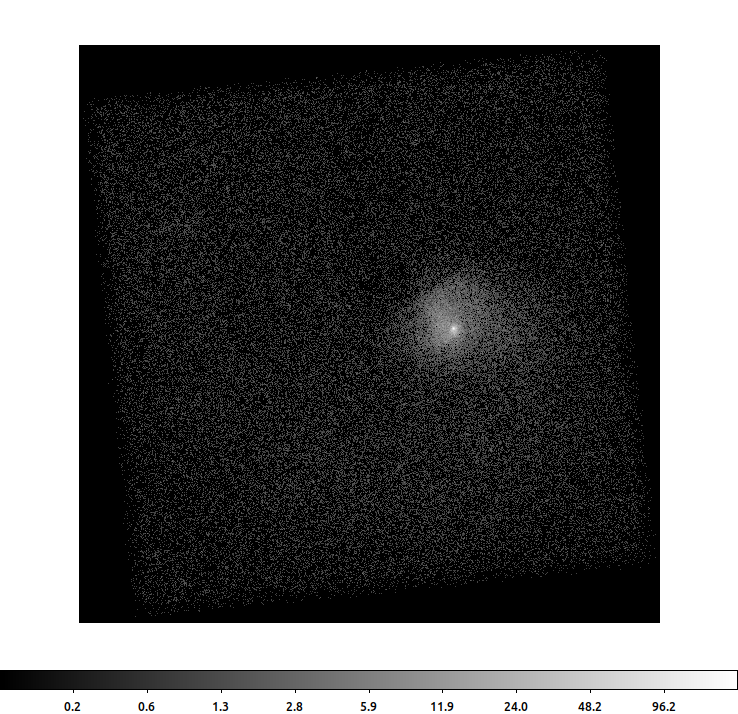### Figure 5: After point sources removed

The broad band image of NGC7618 after the point sources have been removed.

```unix% ds9 ngc7618.filled -block to 2
```

The region with the overlapping regions is shown in Figure 6

### Figure 6: Overlapping source region after point sources removed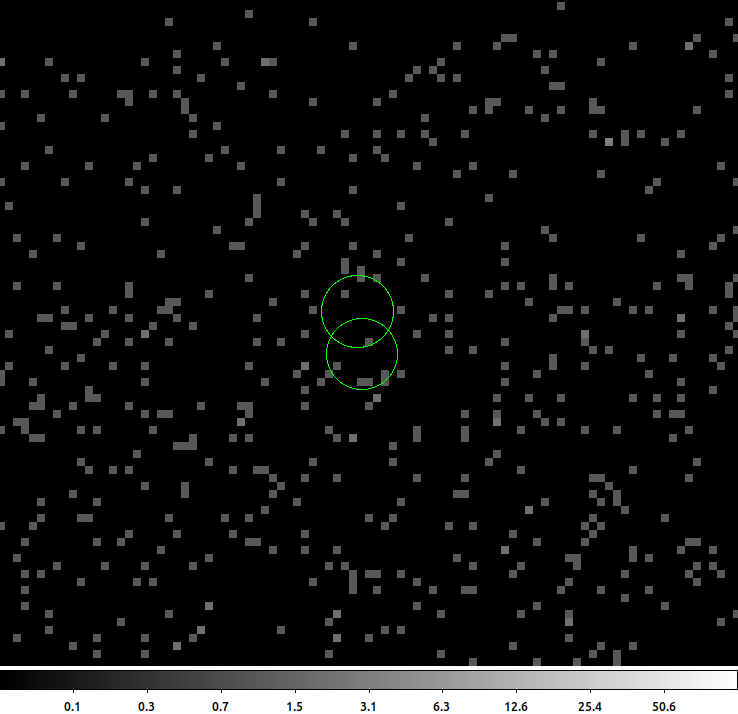### Figure 6: Overlapping source region after point sources removed

The broad band image of NGC7618 after the point sources have been removed zoomed into the region where the sources overlap. The pixels in the overlapping region area have also been filled.

```unix% ds9 ngc7618.filled -zoom to 8 \
-pan to 4003 4540 physical \
```

Our goal is to create a mean energy map, but to compute the mean we need a reasonable number of counts. The mean is easily biased by a few outliers when dealing with low counts. We could try to simply bin the image with a large bin size but that may still leave some low count regions. Instead we choose to use one of the adaptive binning tools in CIAO: dmradar. It works by recursively dividing an annulii into quadrants until dividing it any further would result in the signal-to-noise ratio falling below some threshold.

```unix% punlearn dmradar

snr=7.071 xcenter=3976 ycenter=4057 method=4 \
shape=pie router=750 rinner=4 minradius=2 minangle=1 \
mode=h clob+
```

Since we did not supply an estimate of the uncertainties, the signal-to-noise ratio is computed as the square-root of the number of counts. So in this example snr=7.071 corresponds to a minimum of 50 counts. The output counts image, ngc7618.abin, is shown in Figure 7.

### Figure 7: Adaptively binned image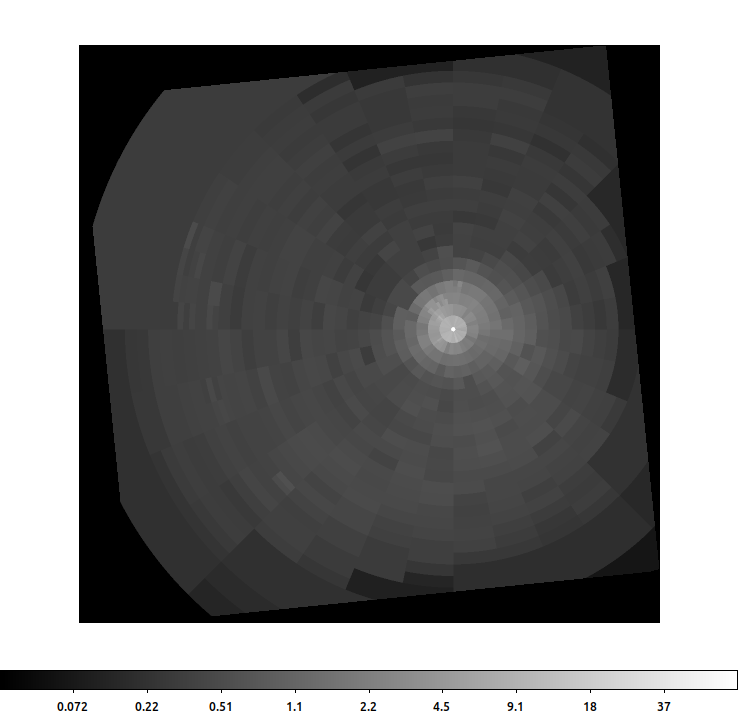### Figure 7: Adaptively binned image

Adaptively binned image of NGC7618. Each bin contains at last 50 counts (broad band).

`unix% ds9 ngc7618.abin -zoom to 0.5`

While the counts are interesting, for this thread the output map file, outmaskfile, is needed.

## Creating Mean Energy Map

### Using statmap

The dmradar outmaskfile file contains a map where the pixel values indicate which group each pixel belongs to. We will use this file to compute the mean energy. This could be replaced with same output from dmnautilus or any number of other adaptive binning routines available in the community.

Before we compute the mean energy of the events, we need to be sure that we only include the events associated with the diffuse emission. That is we need to exclude the events of the point-like sources from the event file. Using the trick shown here, we invert the region file created by psfsize_src and use it to remove the point sources from the event file

```unix% cat << EOM | python
from region import CXCRegion,field
out=field()-CXCRegion("psfsize.src")
out.write("exclude.src", fits=True, clobber=True)
EOM

unix% dmcopy "16014/repro/acisf16014_repro_evt2.fits[sky=region(exclude.src)]" no_point_srcs.evt clob+
```

We then use the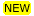statmap tool to compute the mean of the energy column value in the event file.

```unix% punlearn statmap
unix% statmap infile=no_point_srcs.evt"[ccd_id=7,energy=500:7000]" \
mapfile=ngc7618.map \
outfile=ngc7618_median_energy.map \
column=energy statistic=mean clob+
```

The results are shown in Figure 8. The center of the diffuse emission has lower mean energy than the surrounding events (ie suggesting a cool core) and there are other structures extending to the South of the object. Using these quick look data we can see that there is potentially interesting spatially/spectral properties worth further investigations.

### Figure 8: Mean energy map created with statmap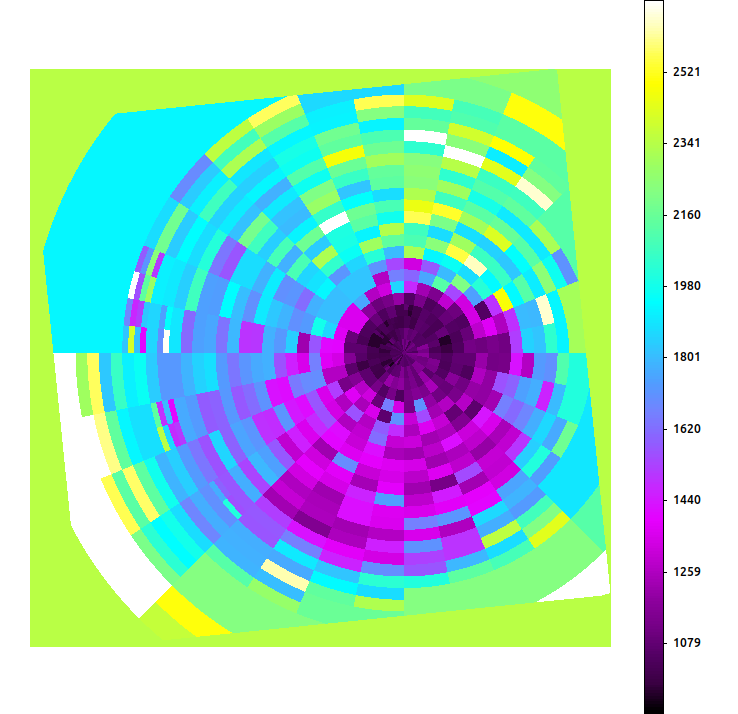### Figure 8: Mean energy map created with statmap

This is the mean energy map created using the statmap tool for the broad band events. The center of the diffuse emission has lower mean energy than the surrounding events and other structures are visible to the South of the source. The units of the energy in the event file are "eV" , so the units of this mean energy map is also eV.

```unix% ds9 ngc7618_median_energy.map -zoom to 0.5 \
-scale linear -scale limits 900 2700 \
-colorbar vertical
```Overlaying grid map boundaries

Sometimes it can be useful to display the map grid boundaries on top of the mean energy map (or even the original counts image). The output map file from dmradar does contain a REGION extension that can be loaded into ds9 by specifying the correct extension

```unix% ds9 ngc7618_median_energy.map -region "ngc7618.map[region]"
```

However, not all adaptive binning routines produce a region file, and in general the regions can be complex (eg contour based algorithms may have a polygon with many hundreds or thousands of sides).

There is a simple way to display the map boundaries using ds9's mask feature. This works for any map file created by any algorithm. First we have to recognize that the map values for each region are unique integer values. What we are interested in is the boundary between these groups of equal values. Topologically, this is the same as where the gradient is non-zero; that is we are only interested in pixels where the values change.

We can compute the gradient of the map file using aconvolve. We just need to convolve the image with a variation of the Laplace gradient operator.

```unix% punlearn aconvolve
unix% aconvolve ngc7618.map"[opt type=i4]" ngc7618.grid "txt:((1,1,1),(1,-8,1),(1,1,1))" norm=none mode=h clob+
```

This convolution kernel is a good choice here because we do not care about the orientation of the gradient, only the magnitude. Even more, we really only care if the magnitude is zero or non-zero.

We can now display the map grid boundaries on top of the mean energy map using ds9's mask option as shown in Figure 9.

### Figure 9: Mean energy map with grid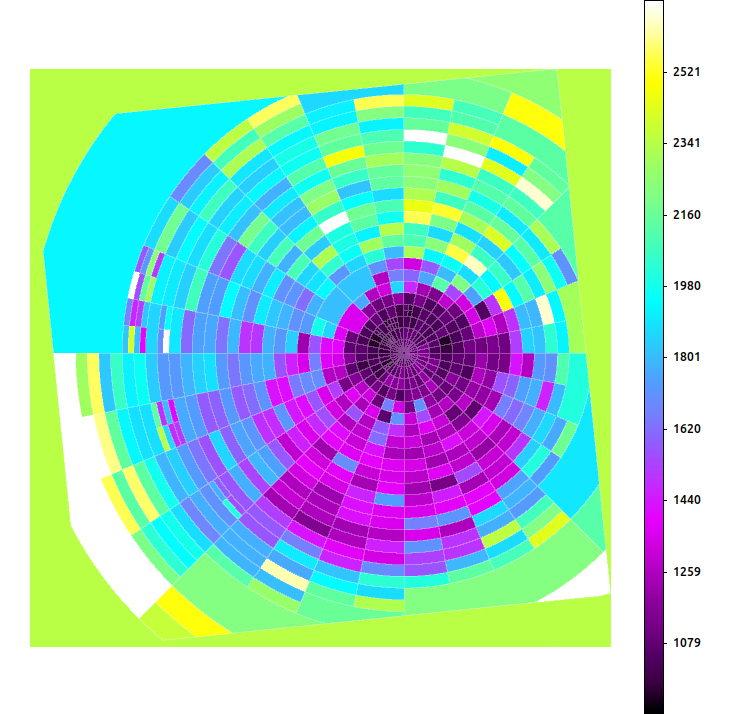### Figure 9: Mean energy map with grid

This is the same as Figure 8 but overlaid with the gradient of the mask file, ie a map of the grid boundaries.

```unix% ds9 ngc7618_median_energy.map -zoom to 0.5 \
-scale linear -scale limits 900 2700 \
-colorbar vertical
```

The transparency of the mask can be adjusted, but the color of the mask cannot be after it has been loaded. Multiple masks can be added with several options to control how the overlapping regions are rendered.

As it's name suggests, statmap can compute several different statistics including: mean, median, min, max, and count. We specified a threshold of 50 counts (snr=7.071) when we ran dmradar. We can use the count option to verify that each region indeed does contain at least 50 counts.

```unix% punlearn statmap
unix% statmap infile=no_point_srcs.evt"[ccd_id=7,energy=500:7000]" \
mapfile=ngc7618.map \
outfile=ngc7618_count.map \
column=energy stat=count clob+
```

The output count map is shown in Figure 10.

### Figure 10: statmap number of counts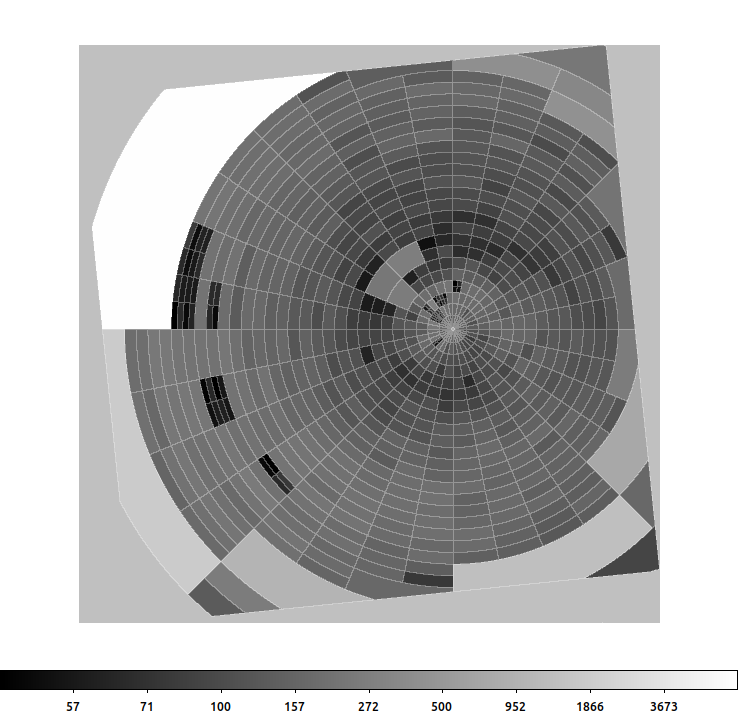### Figure 10: statmap number of counts

This image is the number of counts in each map section. The pixel values are the number of counts in each map segment.

```unix% ds9 ngc7618_count.map -zoom to 0.5 \
-scale log
```

Note the use of zoom rather than block here. In this usage we do not want to bin the data but simply zoom out (ie down sample the image).

We can also check the count map with dmstat to verify that we indeed have a minimum of 50 counts in each region:

```unix% dmstat ngc7618_count.map cen- sig- med-
count_energy
min:        50                 @:        ( 3621 3767 )
max:        7279               @:        ( 3275 4057 )
mean:        936.8222465
sum:        1049684033
good:        1120473
null:        222799
```

### Using mean_energy_map

Users can also create a mean energy map using the mean_energy_map script. This tool uses the eff2evt tool to compute the mirror effective area and detector efficiency for each event based on the event's energy. It then computes an average of all the events in a binned image by weighting the events' energy by the their spectral response.

mean_energy_map is run using the same input event file that we used with statmap, with the point sources removed

```unix% punlearn mean_energy_map
unix% mean_energy_map no_point_srcs.evt"[ccd_id=7,energy=500:7000]" \
outfile=ngc7618_me.map \
bin=8 clob+
```

The bin parameter is used to control the bin size used to create the mean energy map, and thus controls the number of events used when computing the mean value. The results are shown in Figure 11. The variable number of counts in each bin generally produces a noisy image (left frame) and it requires some additional smoothing to be able to make out any spatial features (right frame).

### Figure 11: Mean energy map created with mean_energy_map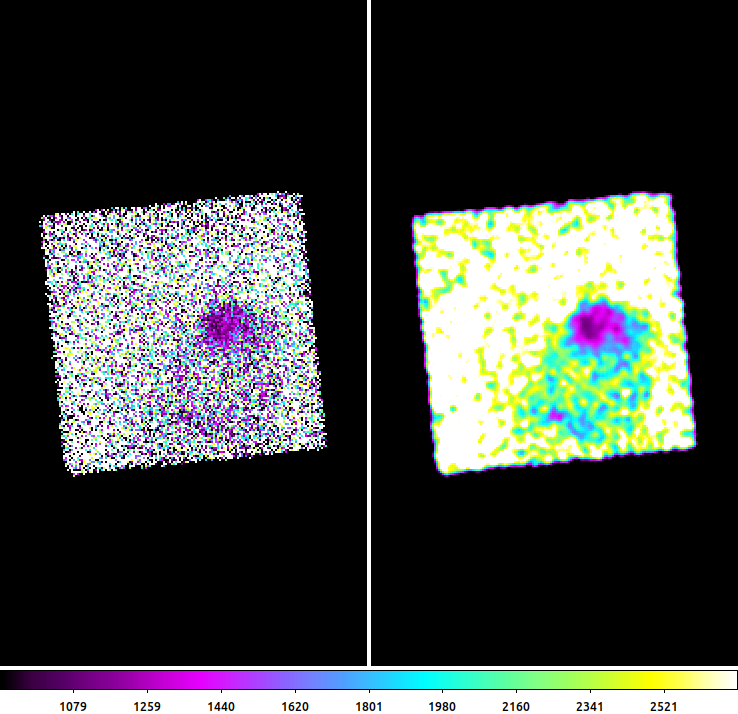### Figure 11: Mean energy map created with mean_energy_map

The output from the mean_energy_map script. (Left) the raw output from mean_energy_map. The pixel values are in units of eV. The image is quite noisy, but the lower energies associated with the core of the diffuse emission is clear. (Right) the same data but smoothed by ds9 (Gaussian, 1.5pixel sigma). The spatial features in the core are better resolved and additional structure to the South is more evident.

```unix% ds9 -view multi no\
ngc7618_me.map -zoom to 2 \
-pan to 3809 4048 physical \
-scale linear -scale limits 900 2700 \
-colorbar vertical \
ngc7618_me.map -zoom to 2 \
-pan to 3809 4048 physical \
-scale linear -scale limits 900 2700 \
-colorbar horizontal \
-smooth
```

There are pro's and con's to using both scripts. The mean_energy_script does take the detector+mirror efficiency into account; though it can only account for events that are actually detected, and the efficiency generally changes slowly across the detector so the relative difference between adjacent bins/pixels is small. statmap does not take the detector efficiency into account, but does allow arbitrary adaptive bin maps to be used, can compute either the mean or median, which may be a more useful statistic for some use cases, and can be used with arbitrary columns in the event file (eg can also create a mean time map).

## Conclusions

The mean energy maps created with these tools are not replacements for temperature maps created by fitting spectra in each of the map regions. However, these maps can provide a quick look at the data to allow users to adjust/optimize settings (eg adaptive binning parameters, energy bands, etc), before investing the time needed to compute true spectral fit based temperature maps.

## History

 05 Jan 2023 First publication of this thread.